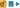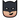# Architecture Outline : Machine Learning AcceleratorYou can find a good introduction and motivation statement to this series of articles on the introduction page. If you already have a good idea about machine learning and a basic understanding of what FPGAs are. Read on!

Part Zero - The Introduction

Part One - The Architecture Outline

Part Two - The Convolution Engine

Part Three - The Activation Function

Part Four - The Pooling Unit

Part Five - Adding fixed-point number support to our design

Part Six - Generating data and automating the Verification Process

Part Seven - Creating a multi-layer neural network in hardware.

## Overview

If we take a look at the architecture of some of the most commonly deployed Convolutional neural networks, we can easily observe that the following are the most common steps/operations repeatedly laid out throughout the network. It is true that present day networks have become extremely complex with all sorts of exotic operations but the following operations form the bedrock for modern day Deep Learning and have brought us a log of progress in this area. I shall definitely add several more nuanced operations to this series as we progress in the project.

For the uninitiated, do skim through this blog post for an excellent introduction on CNNs.

• 2-D Convolution
• Activation Function (Tanh / ReLu)
• Pooling (Max / Average)

## 2-D Convolution

This is probably the first mathematical operation that comes to mind when we think of a Convolutional Neural Network. A 2D convolution is an operation that takes a kernel (or window or matrix) of weights and modifies the input image (or feature map or activation map) in a certain fashion using these weights (the values in this matrix). Read this beautifully written blog post to understand everything about convolutional neural networks and the 2D convolution operation they use.

## Activation Function

An Activation function is introduced between various mathematically linear operations in a neural network with the intention of introducing a non-linearity to the entire network. Without it, the whole neural network would simplify down to a single linear function and we all know that such a function is not good enough to model anything complex, forget something like image recognition.

There have been a variety of functions used for this purpose in the literature, but the most commonly (and first) used ones would be the ReLu ( Rectified Linear Unit) and the Tanh (Hyperbolic Tangent) functions. This is quite evident from the article linked above which summarizes some of the most popular CNN architectures in research.

We shall be implementing both these functions in hardware but our design can only be meaningfully tested when we are done adding fixed-point number support to our design later in this article.

## Pooling

Pooling is essentially a 'down-sampling' operation aimed at reducing the number of parameters and complexity as the data propagates in the network. This process involves running a 'pooling window' over the input and reducing the size of the input using some algorithm. By far the most common algorithms are the Max - Pooling and the Average - Pooling.

Max Pooling - In this method, we only retain the maximum of all the values that are falling into the pooling window and discard the others.

Average Pooling - In this method the average value of all the elements lying inside the pooling window is calculated and that value is retained instead of all the values.

The thing to be noted here is that we need to design the pooler using the same streaming architecture as the convolver if we are achieve the maximum efficiency in the design.

PREVIOUS:The Introduction

NEXT:The convolution engine### Batman

I'm Batman, a silent nerd and a watchful engineer obsessed with great Technology. Get in touch via the Discord community for this site

Like what you are reading? Let me send the latest posts right to your inbox!

Free. No spam. Unsubscribe anytime you wish.